# Projectile Motion

## Projectile Motion

### Basic Concept

1. Projectile motion is a form of motion in which an object or particle (called a projectile) is thrown with some initial velocity near the earth's surface, and it moves along a curved path under the action of gravity alone.
2. It is an example of two dimensional motion with constant acceleration.
3. If $F$ is constant then $a$ is constant and when force is oblique direction with initial velocity , the result is parabolic path.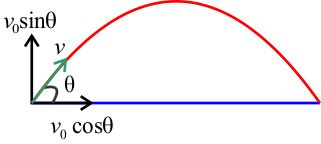4. Projectile motion is considered as two simultaneous motion in mutually perpendicular directions which are completely independent from each other i.e., horizontal motion and vertical motion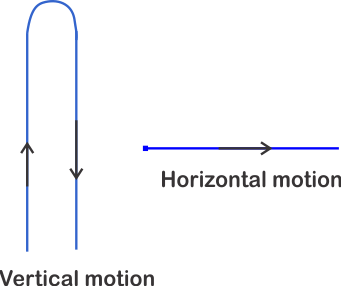### Projectile thrown at an angle with horizontal

So we have seen our basics let us now learn the projectile motion in little more detail about Projectile thrown at an angle with horizontal. There are certain approximate assumptions we make while studying projectile motion and they are
1. We ignore the frictional resistance due to air.
2. The effect due to rotation of earth and the curvature of earth is negligible.
3. The acceleration due to gravity is constant in magnitude and direction at all points during the motion of projectile.
• Projectile motion is a special case of motion in two dimension when acceleration of particle is constant in both magnitude and direction.
• An object is referred as projectile when it is given an initial velocity which subsequently follows a path determined by gravitational forces acting on it. For example bullet fired from the rifle javelin thrown by the athlete etc.
• Path followed by a projectile is called its trajectory.
• In this section we will study the motion of projectile near the earth surface. We would be neglecting the air resistance.
• Acceleration acting on a projectile is constant which is acceleration due to gravity (g=9.81 m/s) directed along vertically downward direction.
• We shall treat the projectile motion in a Cartesian co-ordinates system taking y axis in vertically upwards direction and x axis along horizontal directions
• Now x and y components of acceleration of projectile is
${a_x} = 0$ and ${a_x} = 0$
Since acceleration in horizontal direction is zero, this shows that horizontal component of velocity is constant and vertical motion is simply a case of motion with constant acceleration.
• Suppose at time $t=0$ object is at origin of co-ordinate system and velocity components ${v_{0x}}$ and ${v_{0y}}$. From above components of acceleration are ${a_x} = 0$ and ${a_y} = - g$. From equation (11) and (12) in the previous section components of position and velocity are
$x = {v_{0x}}t$                                          (14a)
${v_x} = {v_{0x}}$                                           (14b)
and ${v_y} = {v_{0y}} - gt$                                          (15b)
$y = {v_{0y}} - \frac{1}{2}g{t^2}$                                          (15a)
• Figure below shows motion of an object projected with velocity ${v_0}$ at an angle ${\theta _0}$ .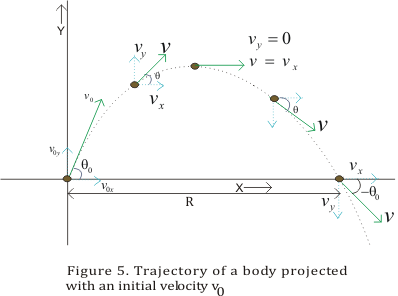• In terms of initial velocity ${v_0}$ and angle ${\theta _0}$ components of initial velocity are
${v_{0x}} = {v_0}cos{\theta _0}$                                          (16a)
${v_{0y}} = {v_0}sin{\theta _0}$                                           (16b)

• Using these relations in equation 14 and 15 we find
x=(v0cosθ0)t                                          (17a)
y=(v0sinθ0)t-(1/2)gt2                                           (17b)
vx=v0cosθ0                                           (17c)
vy=v0sinθ0-gt                                           (17d)

Above equations describe the position and velocity of projectile as shown in fig 5 at any time t.

(A)Equation of Path of projectile(Trajectory)
• From equation 17a
t=x/v0cosθ0
now putting this value of t in equation 17b,we find
y=(tanθ0 )x-[g/2(v0cosθ0)2]x2                                           (18)

In equation (18),quantities θ0,g and v0 are all constants and equation (18) can be compared with the equation
y=ax-bx2
where a and b are constants

• This equation y=ax-bx2 is the equation of the parabola.From this we conclude that path of the projectile is a parabola as shown in figure 5
B) Time of Maximum height
• At point of maximum height vy=0.Thus from equation (17d)
vy=v0sinθ0-gt
0=v0sinθ0-gt -
or tm=v0sinθ0/g                                          (19)
• Time of flight of projectile which is the total time during which the projectile is in flight can be obtained by putting y=0 because when projectile reaches ground ,vertical distance travelled is zero.This from equation (17b)

tf=2(v0sinθ0)/g                                          (20)
or
tf=2tm
• Maximum height reached by the projectile can be calculated by substituting t=tm in equation 17b
y=Hm=(v0sinθ0)(v0sinθ0/g)-(g/2)(v0sinθ0/g)2
or
Hm=v02sin2θ0/2g                                          (21)
(C) Horizontal Range of Projectile
• Since acceleration g acting on the projectile is acting vertically ,so it has no component in horizontal direction.
• So, projectile moves in horizontal direction with a constant velocity v0cosθ0. So range R is
R=OA=velocity x time of flight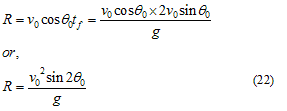• Maximum range is obtained when sin2θ0=1 or θ0=450. Thus when θ0=450 maximum range achieved for a given initial velocity is (v0)2/g.

### Projectile Motion of an object fired Horizontally from the top of the tower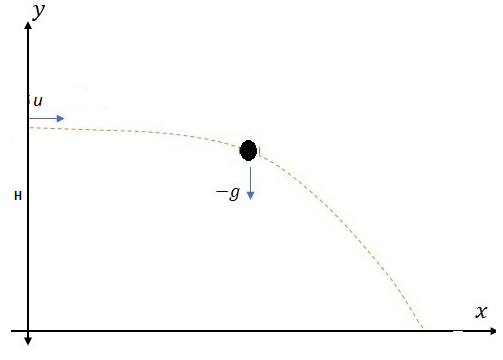• We shall treat the projectile motion in a Cartesian co-ordinates system taking y axis in vertically upwards direction and x axis along horizontal directions
• Now x and y components of acceleration of projectile is
$a_x=0$ and $a_y=-g$
• Suppose at time t=0 object is at height h on the y-axis of co-ordinate system and is fired with horizontal velocity u.
So, components of position and velocity are
$x= ut$ -(1)
$v_x= u$
and
$y= 0 – \frac {1}{2} gt^2$ -(2)
$v_y=-gt$
Substituting the value of t from (1) in (2)
$y=-\frac {1}{2}g(\frac {x}{u})^2$
Or
$y=-\frac {(gx^2)}{2u}$
This is an equation of a parabola
• Velocity of the object at any instant is given by
$v_x= u$ and $v_y =-gt$
Magnitude of Velocity
$v=\sqrt {(u^2+g^2 t^2 )}$

### Impact of air resistance on the motion of the projectile.

So far we have assumed that air resistance has no effect on the motion of the projectile.Now we know Friction, force due to viscosity, air resistance are all dissipative forces. In the presence of any of such forces opposing motion, any object will lose some part of its initial energy and consequently, momentum too. Thus, a projectile that traverses a parabolic path would certainly show deviation from its idealised trajectory in the presence of air resistance. It will not hit the ground with the same Speed with which it was projected from it. In the absence of air resistance, the x-component of the velocity remains constant and it is only the y-component that undergoes a continuous change. However, in the presence of air resistance, both of these would get affected. That would mean that the range would be less than the one given above Maximum height attained would also be less than that predicted by above. The mathematics of the motion with air resistance is quite complicated (especially if you consider the change in the shape and/or surface of a projectile and the variation of the density of the air with height) but the following diagrams try to simplify things by showing generally how air resistance affects trajectory of a projectile.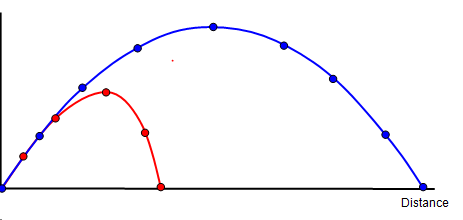When we use a phrase like ?neglect air resistance?, we imply that the change in parameters such as range, height etc. is much smaller than their values without air resistance. The calculation without air resistance is much simpler than that with air resistance

## Concept Map's for projectile motion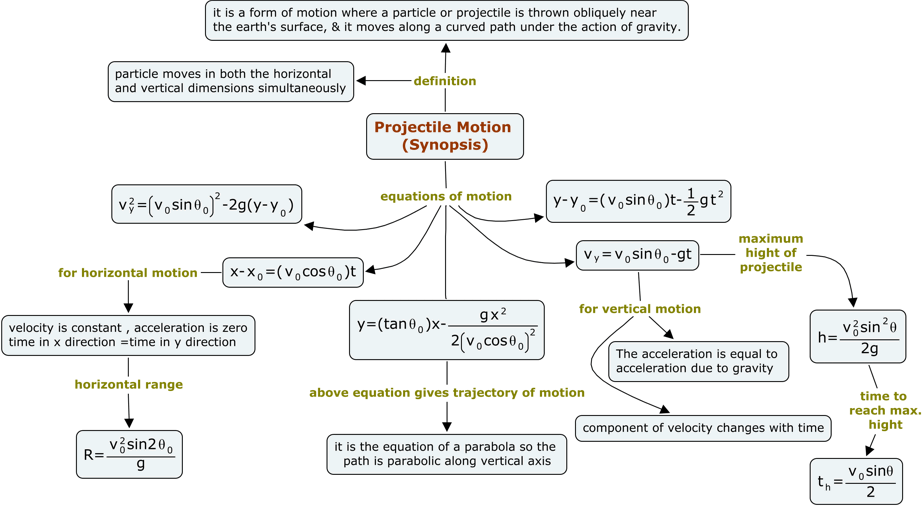## Solved Examples

Question 1
A football is kicked from horizontal at speed 18m/s and angle 30°. Find
1. How much later does it hit the ground?
2. How much height it goes Up?
3.How far from its initial does it hit the ground?
Solution
Here $v_0=18$ m/s and $\theta _0 =30$ °
Time of Flight is given by
$T= \frac {2v_0 \sin \theta_0}{g}=\frac {2 \times 18 \times .5}{10}=1.8$ sec
Height to be which goes Up
$H=\frac {v_0^2 \sin^2 \theta _0}{2g}=\frac {18 \times 18 \times .5 \times .5}{20}=4.05$ m
Range is given by
$R=\frac {v_0^2 \sin 2 \theta _0}{g}=\frac {18 \times 18 \times .866}{10}=28.04$ m

Question 2
A ball is thrown horizontally at velocity 22.2 m/s from the roof of tho tower of Height H. It is found that it land on the ground at a distance 36 m from the ground . find H
Solution
Here u=22.2 m/s and R=36 m
Now
R=ut
or
T= R/u= 36/22.2=1.621 sec
Now
$H= \frac {1}{2} gt^2= .5 \times 10 \times (1.621)^2=13.14$ m

Question 3
Prove that maximum horizontal range is four times the maximum height attained by the projectile when fired at an incliniation so as to have the maximum Range.
Solution
We know that
$R=\frac {v_0^2 \sin 2 \theta _0}{g}$
which is maximum when $\sin 2 \theta _0=1$ i.e $\theta_0=45^0$
Maximum Range will be
$R=\frac {v_0^2 }{g}$
Now Maximum height attained
$H=\frac {v_0^2 \sin^2 \theta _0}{2g}=\frac {v_0^2 \sin^2 45}{2g}=\frac {v_0^2}{4g}= \frac {R}{4}$
Therefore R=4H